# Autocorrelation

The autocorrelation of one magnitude refers to the temporal correlation of a magnitude with itself. The magnitude may be scalar:$c(t)=\langle a(0) a(t) \rangle,$

or vectorial, in which case the scalar product is taken: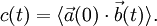$c(t)=\langle \vec{a}(0)\cdot\vec{b}(t) \rangle.$

These correlations typically decay exponentially at long times: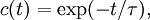$c(t)=\exp(-t/\tau),$

with a decay, or relaxation, time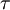$\tau$. This holds if the underlying process is Markovian, and exceptions are known to occur, even in equilibrium classical fluids: the velocity autocorrelation function (see diffusion) is known to present a long tail (power-law) decay.

A different definition of the decay time would be the time integral of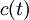$c(t)$: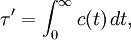$\tau'=\int_0^\infty c(t) \,dt ,$

which coincides with the previous one if the decay is purely exponential. Since this is not the case at short times, the two times will be similar but different. This later definition seems to be more related to times experimentally measurable.